# `sklearn.linear_model`.LinearRegression¶

class `sklearn.linear_model.``LinearRegression`(fit_intercept=True, normalize=False, copy_X=True, n_jobs=1)[source]

Ordinary least squares Linear Regression.

Parameters: fit_intercept : boolean, optional whether to calculate the intercept for this model. If set to false, no intercept will be used in calculations (e.g. data is expected to be already centered). normalize : boolean, optional, default False If True, the regressors X will be normalized before regression. This parameter is ignored when fit_intercept is set to False. When the regressors are normalized, note that this makes the hyperparameters learnt more robust and almost independent of the number of samples. The same property is not valid for standardized data. However, if you wish to standardize, please use preprocessing.StandardScaler before calling fit on an estimator with normalize=False. copy_X : boolean, optional, default True If True, X will be copied; else, it may be overwritten. n_jobs : int, optional, default 1 The number of jobs to use for the computation. If -1 all CPUs are used. This will only provide speedup for n_targets > 1 and sufficient large problems. coef_ : array, shape (n_features, ) or (n_targets, n_features) Estimated coefficients for the linear regression problem. If multiple targets are passed during the fit (y 2D), this is a 2D array of shape (n_targets, n_features), while if only one target is passed, this is a 1D array of length n_features. residues_ : array, shape (n_targets,) or (1,) or empty Sum of residuals. Squared Euclidean 2-norm for each target passed during the fit. If the linear regression problem is under-determined (the number of linearly independent rows of the training matrix is less than its number of linearly independent columns), this is an empty array. If the target vector passed during the fit is 1-dimensional, this is a (1,) shape array. New in version 0.18. intercept_ : array Independent term in the linear model.

Notes

From the implementation point of view, this is just plain Ordinary Least Squares (scipy.linalg.lstsq) wrapped as a predictor object.

Methods

 `decision_function`(\*args, \*\*kwargs) DEPRECATED: and will be removed in 0.19. `fit`(X, y[, sample_weight]) Fit linear model. `get_params`([deep]) Get parameters for this estimator. `predict`(X) Predict using the linear model `score`(X, y[, sample_weight]) Returns the coefficient of determination R^2 of the prediction. `set_params`(\*\*params) Set the parameters of this estimator.
`__init__`(fit_intercept=True, normalize=False, copy_X=True, n_jobs=1)[source]
`decision_function`(*args, **kwargs)[source]

DEPRECATED: and will be removed in 0.19.

Decision function of the linear model.

Parameters: X : {array-like, sparse matrix}, shape = (n_samples, n_features) Samples. C : array, shape = (n_samples,) Returns predicted values.
`fit`(X, y, sample_weight=None)[source]

Fit linear model.

Parameters: X : numpy array or sparse matrix of shape [n_samples,n_features] Training data y : numpy array of shape [n_samples, n_targets] Target values sample_weight : numpy array of shape [n_samples] Individual weights for each sample New in version 0.17: parameter sample_weight support to LinearRegression. self : returns an instance of self.
`get_params`(deep=True)[source]

Get parameters for this estimator.

Parameters: deep : boolean, optional If True, will return the parameters for this estimator and contained subobjects that are estimators. params : mapping of string to any Parameter names mapped to their values.
`predict`(X)[source]

Predict using the linear model

Parameters: X : {array-like, sparse matrix}, shape = (n_samples, n_features) Samples. C : array, shape = (n_samples,) Returns predicted values.
`residues_`

DEPRECATED: `residues_` is deprecated and will be removed in 0.19

Get the residues of the fitted model.

`score`(X, y, sample_weight=None)[source]

Returns the coefficient of determination R^2 of the prediction.

The coefficient R^2 is defined as (1 - u/v), where u is the regression sum of squares ((y_true - y_pred) ** 2).sum() and v is the residual sum of squares ((y_true - y_true.mean()) ** 2).sum(). Best possible score is 1.0 and it can be negative (because the model can be arbitrarily worse). A constant model that always predicts the expected value of y, disregarding the input features, would get a R^2 score of 0.0.

Parameters: X : array-like, shape = (n_samples, n_features) Test samples. y : array-like, shape = (n_samples) or (n_samples, n_outputs) True values for X. sample_weight : array-like, shape = [n_samples], optional Sample weights. score : float R^2 of self.predict(X) wrt. y.
`set_params`(**params)[source]

Set the parameters of this estimator.

The method works on simple estimators as well as on nested objects (such as pipelines). The latter have parameters of the form `<component>__<parameter>` so that it’s possible to update each component of a nested object.

Returns: self :

## Examples using `sklearn.linear_model.LinearRegression`¶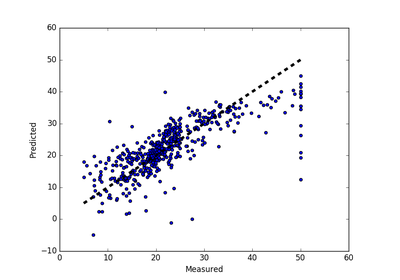Plotting Cross-Validated Predictions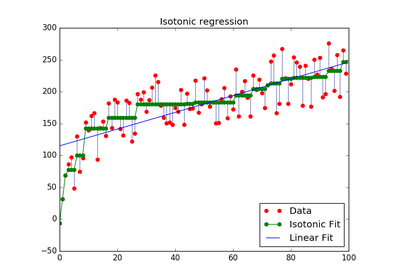Isotonic Regression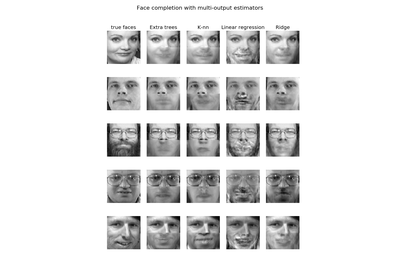Face completion with a multi-output estimators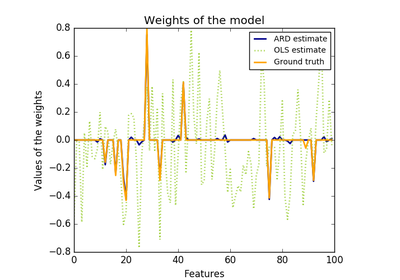Automatic Relevance Determination Regression (ARD)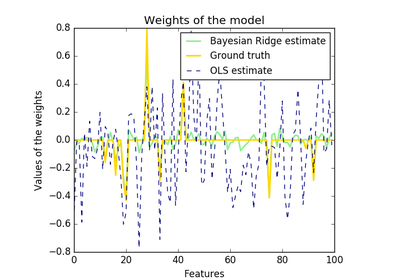Bayesian Ridge Regression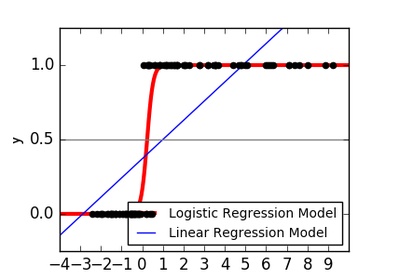Logistic function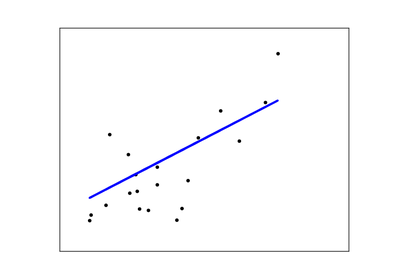Linear Regression Example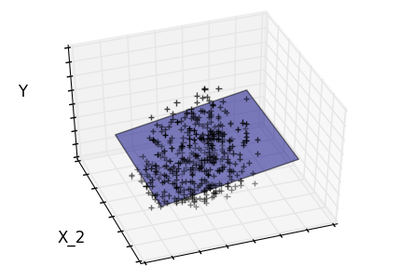Sparsity Example: Fitting only features 1 and 2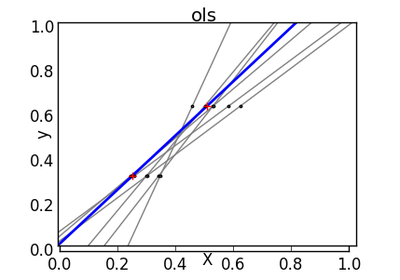Ordinary Least Squares and Ridge Regression Variance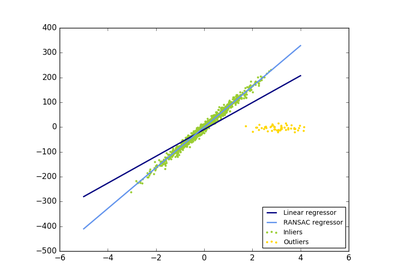Robust linear model estimation using RANSAC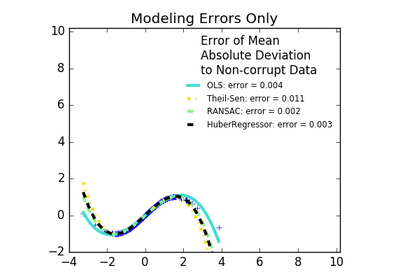Robust linear estimator fitting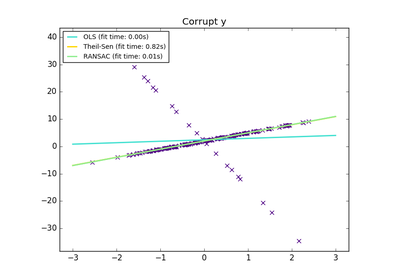Theil-Sen Regression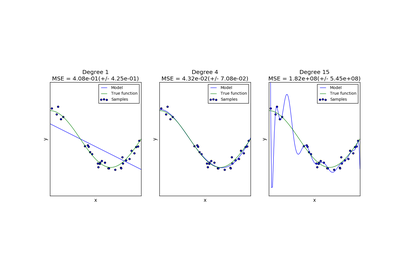Underfitting vs. Overfitting